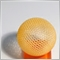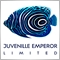# settings97

hi

how can calculate the exact ratio of my potential earnings according to the balance , initial deposit , and leverage of mine and the provider ?

thank youModerator
10441

pinkyy pink:

hi

how can calculate the exact ratio of my potential earnings according to the balance , initial deposit , and leverage of mine and the provider ?

thank you

You could always make an assumption based on these factors, but real signal copying will be very different from these calculations.

Slippage and different trading conditions can alter your final copying results a lot.97

Eleni Anna Branou:

You could always make an assumption based on these factors, but real signal copying will be very different from these calculations.

Slippage and different trading conditions can alter your final copying results a lot.

what in case i assume these factors to be the same for next time ?Moderator
10441

pinkyy pink:

what in case i assume these factors to be the same for next time ?

Then make some standard calculations, like if you have half of signal provider's current balance and same leverage, you could expect about half of his % profit.

If your leverage is smaller or larger, then again calculate these accordingly, for example if your leverage is 1:100 and signal provider's 1:500, you will have 1/5th of signal's profits.

There isn't a fixed formula for all that, if this is what you are looking about.Moderator
10441